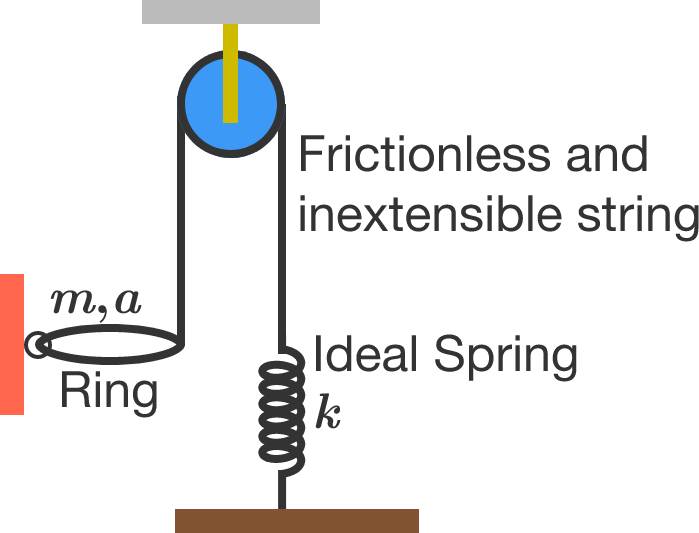# Awesome SHM!A ring of mass $m$ and radius $a$ is connected to an inextensible string which passes over a frictionless pulley. The other end of the string is connected to the upper end of a massless spring of spring constant $k$. The lower end of the spring is fixed to the ground. The ring can rotate in the vertical plane about hinge without any friction. If the horizontal position of the ring is in equilibrium, then the time period of small oscillations is given by $T=2 \pi \sqrt{\frac{im}{bk}}.$

Find $i+b.$

Note: $\gcd(i,b)=1.$

This problem is not original.

×